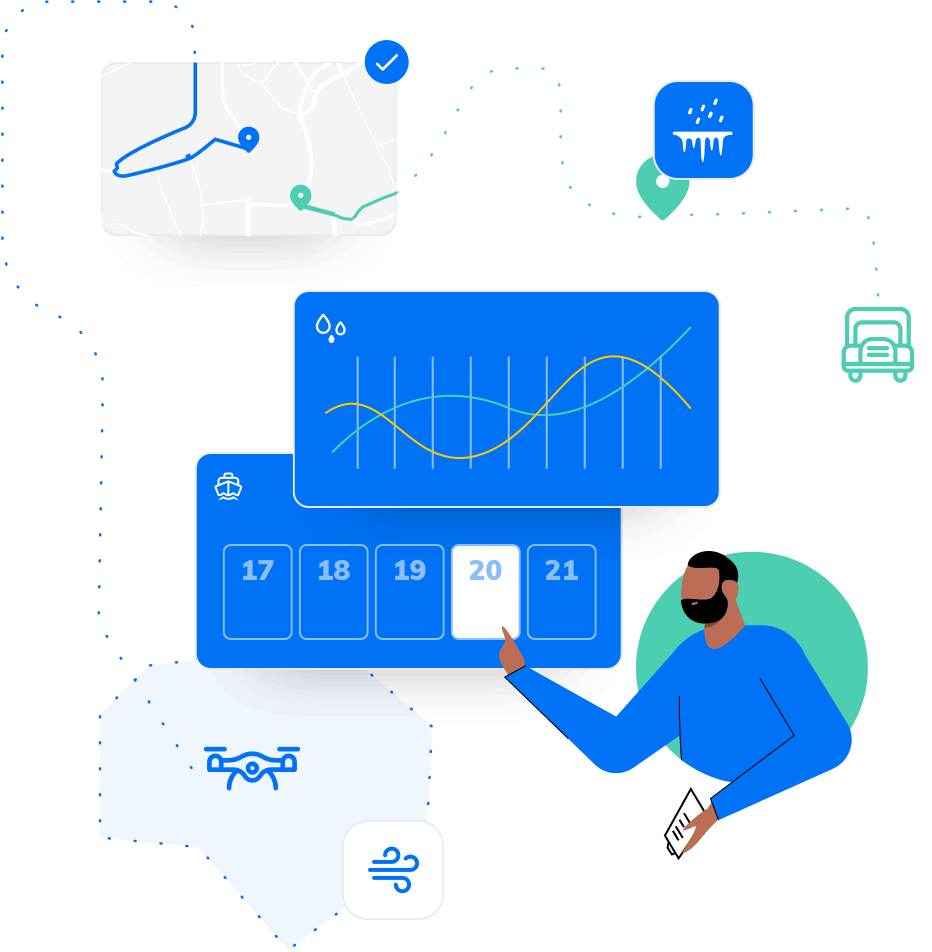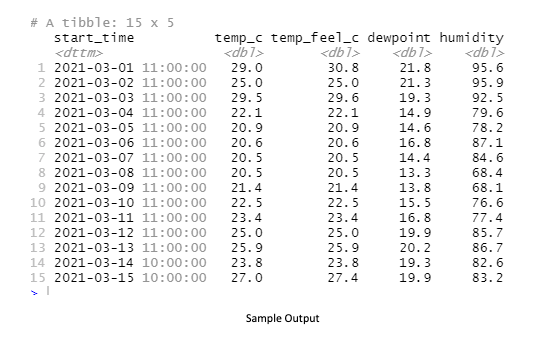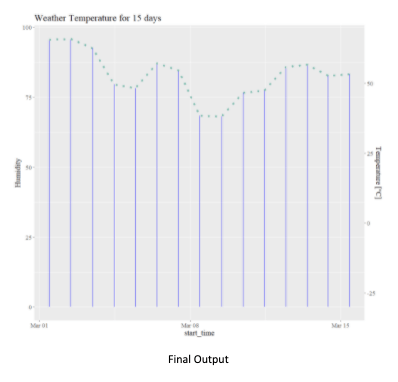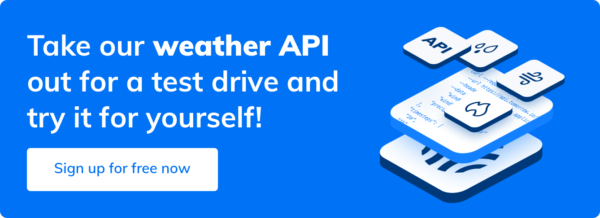Save your seat for ClimaCon 2022 now!# Creating a Weather Data App Using Tomorrow.io API R Package

If you’re in mobile or web application development, chances are you’ve probably coded or experimented with weather applications before. Have you ever thought about statistically showing and plotting weather forecasts? That’s what we’ll focus on in this post, using the Tomorrow.io weather API to give us the hyper-accurate weather data we need.

This guide focuses on using R. So, to follow along you will need to have R and R-Studio installed in your machine.

Here are some packages you need to install at your end:

• Githubinstall – this helps developers install R packages hosted on GitHub. You can install it using the command: install.packages(“githubinstall”).
• Httr – this is a tool for working with HTTP. You can install it using the command: install.packages(“httr”).
• Rtomorrow.io – this is a wrapper for the Tomorrow.io API. You can install it using the command: install.packages(“Rtomorrow.io”).
• Ggplot2 – a library that deals with data visualizations. You can install using this command: install.packages(“ggplot2”).

Once you get your unique API key from Tomorrow.io, you can store and access your secret key in the R application using this code sample:

```#need to have an API for the authentication on Tomorrow API.
Sys.setenv(TOMORROW_API = "")

#in order to access the API key later this the way to do it
print(Sys.getenv("TOMORROW_API"))```

Before you can use the API, you need to know your current location or coordinates in terms of longitude and latitude.  However, you can’t just get your current coordinates; you will need to use some packages to help you.

Here are the steps for getting your current coordinates

1. Install myip is an R package tool that determines your public IP address. You can install it using this command in the console:  githubinstall::gh_install_packages(“myip”).
2. Use the httr library and request http://ip-api.com/ to get the coordinates.
3. Once you have these values ready, you will need to pass these as an argument to the Tomorrow functions. This will be done in a later section.

See the sample code below:

```library(myip) #github package
library(stringr) #string library
library(httr) #httr library

ip <- substr(toString(myip("random")),start =15, stop=28)

#print(ip)

#get our coordinates
url <- gsub(" ","", paste('http://ip-api.com/json/',ip, "?fields=lat,lon"))

#store the results
result <- GET(url)

#store results as parsed
json <- content(result,as="parsed")

#uncomment if you want to see your coordinates
#print(json)
#print(json)```

# Using Tomorrow.io R package

In this section, you need to pass the data that you prepared from the previous code samples, which requires invoking one of the Tomorrow.io wrapper API functions and, in this example, we’re going to use the tomorrow.io_temperature method.

Let us see the example below before we go into the code details.

```library(myip) #github package
library(stringr) #string library
library(httr) #httr library
library(Rtomorrow.io) #tomorrow.io API wrapper library
library(lubridate) #date library

#need to have an API for the authentication on Tomorrow.io API.
Sys.setenv(tomorrow.io_API = "aFK9oVioFuoSJ51CzXm45acAn8pDOfak")

ip <- substr(toString(myip("all")),start =15, stop=28) #if 'random' doesn't work use 'all'

#get our coordinates
url <- gsub(" ","", paste('http://ip-api.com/json/',ip, "?fields=lat,lon"))

#store the results
result <- GET(url)

#store results as parsed
json <- content(result,as="parsed")

start_time <- lubridate::now()
end_time <- st + lubridate::days(15)

#for the argument of timestep 'current','1m','5m','15m','30m','1h','1d' are the only acceptable values.

weather_result <- Tomorrow.io_temperature(api_key = Sys.getenv("tomorrow.io_API"),
lat = as.numeric(json),
long = as.numeric(json),
timestep = '1d',
start_time = start_time,
end_time = end_time)
print(weather_result)```

As you can see from the code sample above, we have passed the required arguments to the tomrrow.io_temperature method, like the time and coordinates and, of course, the API key. It is a straightforward method. However, the next challenge is how we can plot an image to make weather temperature forecasts.

But before that, please see the sample output below.# Using Tomorrow.io R and Plotting the Forecast Temperature

I know you’re excited to see the full sample of our code. So before going even further, let me show the full code sample below.

```library(myip) #github package
library(stringr) #string library
library(httr) #httr library
library(Rtomorrow.io) #Tomorrow API wrapper library
library(lubridate) #date library
library(ggplot2) #graph
library(hrbrthemes)#themes
library(extrafont)

#need to have an API for the authentication on Tomorrow API.
Sys.setenv(TOMORROW_API = "aFK9oVioFuoSJ51CzXm45acAn8pDOfak")

ip <- substr(toString(myip("all")),start =15, stop=28) #if 'random' doesn't work use 'all'

#get our coordinates
url <- gsub(" ","", paste('http://ip-api.com/json/',ip, "?fields=lat,lon"))

#store the results
result <- GET(url)

#store results as parsed
json <- content(result,as="parsed")

start_time <- lubridate::now()
end_time <- st + lubridate::days(15)

#for the argument of timestep 'current','1m','5m','15m','30m','1h','1d' are the only acceptable values.

weather_result <- Tomorrow.io_temperature(api_key = Sys.getenv("tomorrow.io_API"),
lat = as.numeric(json),
long = as.numeric(json),
timestep = '1d',
start_time = start_time,
end_time = end_time)
glimpse(weather_result)

graph_result <- ggplot(data=weather_result, aes(x=start_time, y=humidity, group=1)) +
geom_bar(stat = "identity", color="blue", fill="blue", width =5)+
geom_line( color="#69b3a2", size=2, alpha=0.9, linetype=3) +
scale_y_continuous("Humidity", sec.axis = sec_axis(~ . -30, name = "Temperature [°C]")) +
theme(text=element_text(size=16,  family="serif")) +
ggtitle("Weather Temperature for 15 days")

show(graph_result)```In this final section, by setting the data, x- and y-axis values of the ggplot parameters from the weather_result, we have plotted the data given to us by the Tomorrow.io API forecast. Don’t forget the image results on your R Studio’s “Plots” tab.

# Summary

Today, we have shown how to get started with Tomorrow.io’s API using the R language, and we have tried to demonstrate the following:

•         Showed where to get your API key location via the dashboard.
•         Get your current coordinates via a 3rd party Github package.
•         Talk to Tomorrow.io’s API wrapper for R language to get the temperature.
•         Showed how to plot the weather temperature forecasts using the R language.## How to Deal With Severe Weather Season in the Southeast

### Register for ClimaCon 2021

ClimaCon is a completely free, digital conference. Register below.